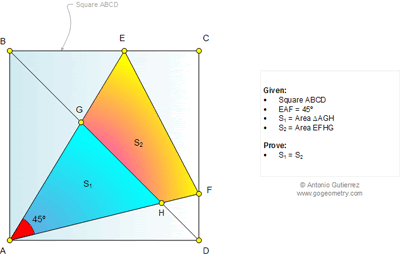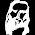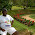## Tuesday, May 13, 2014

### Geometry Problem 1014. Square, Diagonal, 45 Degrees, Angle, Equal Areas, Triangle, Quadrilateral

Level: Mathematics Education, High School, Honors Geometry, College.

Click the figure below to enlarge the figure of problem 1014.1.Since ∠ (EAH)= ∠ (EBH)=45 => ABEH is cocyclic
And ∠ (EHA)= 90 => AEH is a right isosceles triangle
AE/AH=sqrt(2)
Similarly we also have AF/AG= sqrt(2)
Triangle AGH similar to triangle(AFE) with ratio of similarity= sqrt(2)
And area(AEF)=2. Area(AGH)
So S1=S2

2.It suffice to prove that (AE×AF)/(AG×AH) = 2.

Using trigonometry,
Let ∠BAE=α and ∠DAF=β, then α+β=45°.

(AE×AF)/(AG×AH)
= AE/AG × AF/AH
= (1 + BE/AB) × (1 + DF/AD)
= (1 + tanα) × (1 + tanβ)
= 1 + tanα tanβ + tanα + tanβ
= 1 + tanα tanβ + tan(α+β) [1 − tanα tanβ] --- Compound angle formula
= 1 + tanα tanβ + 1 − tanα tanβ
= 2

3.AGFD is cyclic GF is perpendicular to AG. Let AG = GF = a and let GE = b

ABEH is cyclic so AH is perpendicular to EH and AH = EH = (a+b)/sqrt2

S1 = S(AGF) X AH/AF = a^2/2 X (a+b)/sqrt2 / (sqrt2 a)= a(a+b)/4...(1)

S1+S2 = 1/2 a(a+b)...(2)

From (1) and (2) S1 + S2 = 2S1 and hence S1 = S2

Sumith Peiris
Moratuwa
Sri Lanka